ray.c.carpenter

•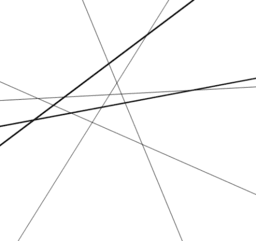Crossings

Activity

ray.c.carpenter

•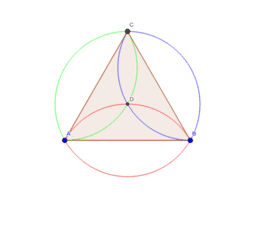Equilateral triangle from a circle

Activity

ray.c.carpenter

•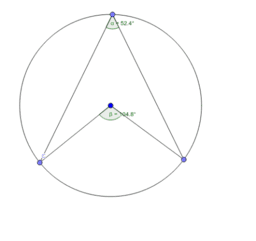Circle Theorems and other geometry

Book

ray.c.carpenter

•Alternative equilateral triangle diagram 2b

Activity

ray.c.carpenter

•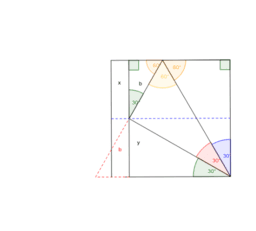Alternative equilateral triangle diagram 2a

Activity

ray.c.carpenter

•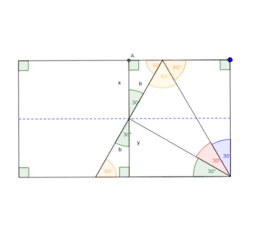Equilateral triangle from a rectangle - diagram 1b

Activity

ray.c.carpenter

•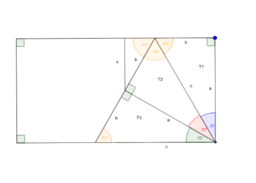Equilateral triangle from a rectangle - diagram 1a

Activity

ray.c.carpenter

•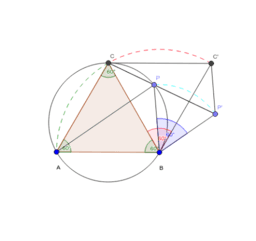van Schooten's theorem extended diagram for proof

Activity

ray.c.carpenter

•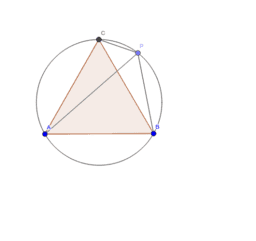van Schooten's theorem start point

Activity

ray.c.carpenter

•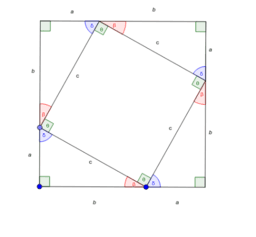Dynamic Pythagoras drawing for proof

Activity

ray.c.carpenter

•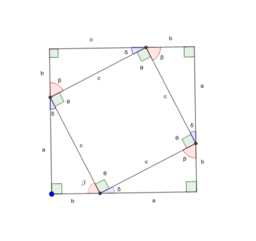Static Pythagoras drawing for proof

Activity

ray.c.carpenter

•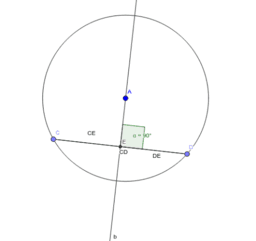Perpendicular from centre to chord

Activity

ray.c.carpenter

•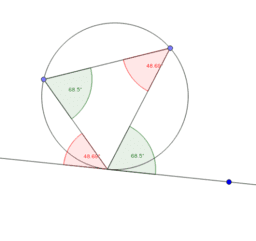Alternate segment

Activity

ray.c.carpenter

•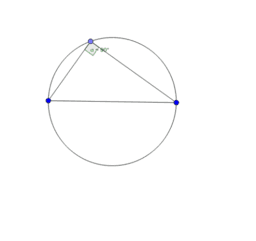Angle in semicircle

Activity

ray.c.carpenter

•Angle at centre and circumference

Activity

ray.c.carpenter

•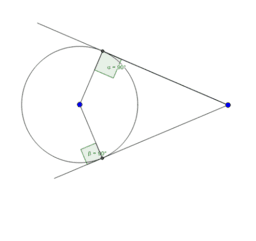Activity

ray.c.carpenter

•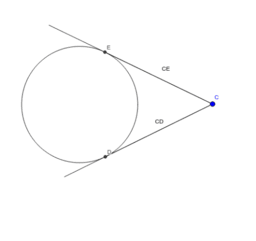Tangents from a point

Activity

ray.c.carpenter

•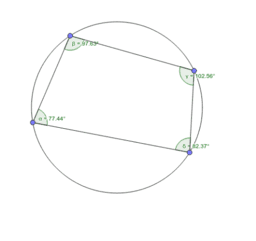Opposite angles in a cyclic quadrilateral

Activity

ray.c.carpenter

•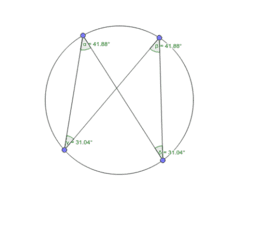Angles in the same segment

Activity

ray.c.carpenter